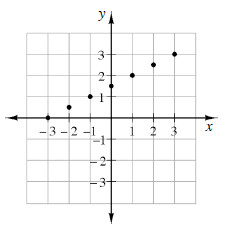### Home > MC1 > Chapter 8 > Lesson 8.2.4 > Problem8-68

8-68.

Below is a graph of seven coordinate points that satisfy the same rule.1. Organize the points by completing the table below.

 $x$ $y$ $–3$ $–2$ $–1$ $0$ $1$ $2$ $3$ $0.5$

For each of the listed $x$-values, find the corresponding $y$-value.

Some of the $y$-values have been put in the table for you. Complete the rest of the table on your own.

 $x$ $y$ $–3$ $–2$ $–1$ $0$ $1$ $2$ $3$ $\color{red}0$ $0.5$ $\color{red}{1.5}$ $\color{red}{2.5}$

1. Name at least two other points that satisfy the same rule as the points in the table.

These points make a line on the graph. What are other points that would fall on the line?